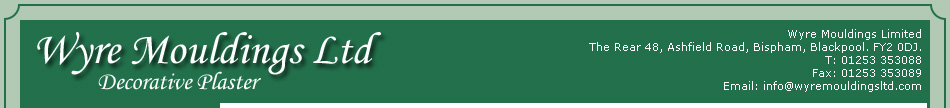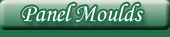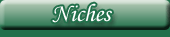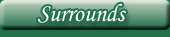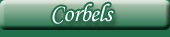# Plaster Cornices

Size Key;

A = Ceiling width.

B = Wall depth.

C = Length.

All measurements are in inches. Images are not to scale.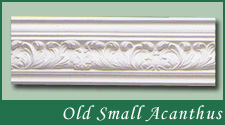A = 4". B = 4.5". C = 120"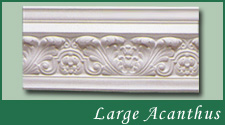A = 5.5". B = 6.25". C = 120"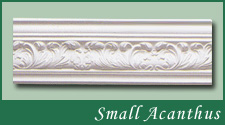A = 4.5". B = 4.25". C = 112"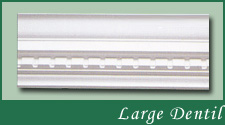A = 5.25". B = 5.5". C = 120"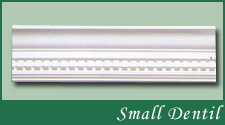A = 3.5". B = 4.25". C = 120"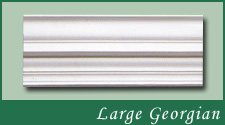A = 5.5". B = 6.25". C = 120"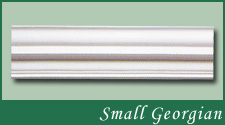A = 3.5". B = 4.5". C = 120"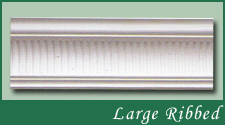A = 5.25". B = 5". C = 120"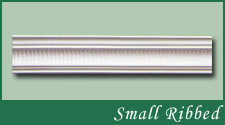A = 2.25". B = 4.25". C = 120"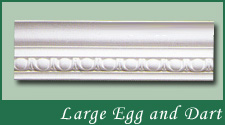A = 4.75". B = 4.75". C = 120"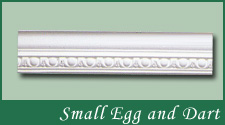A = 3.5". B = 3.75". C = 120"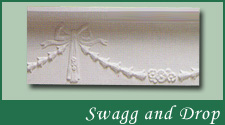A = 2.25". B = 8". C = 120"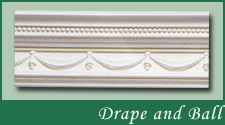A = 3.25". B = 5.5". C = 120"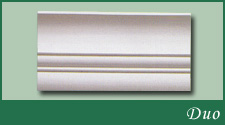A = 6.5". B = 8". C = 114"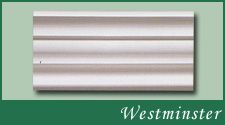A = 6.25". B = 7.5". C = 120"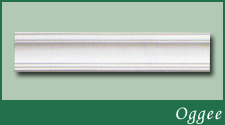A = 4.25". B = 4.25". C = 120"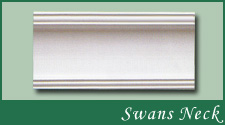A = 7.25". B = 6.5". C = 120"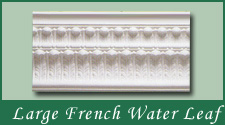A = 6.5". B = 8.25". C = 120"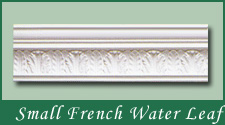A = 3.25". B = 5". C = 118"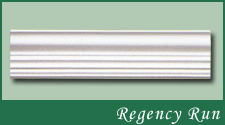A = 3.25". B = 4". C = 117"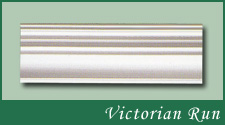A = 4.25". B = 4.75". C = 120"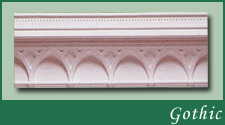A = 5.5". B = 8.25". C = 120"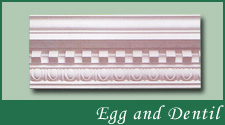A = 7.25". B = 6.5". C = 120"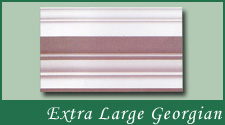A = 8". B = 10". C = 106"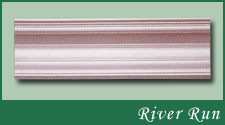A = 7.25". B = 4.25". C = 120"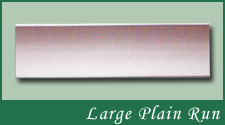A = 4.5". B = 6". C = 120"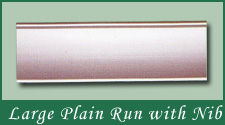A = 6.5". B = 5". C = 120"

NEW THIS YEAR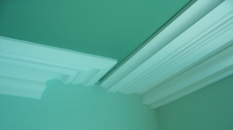Name Here  A = 7.25". B = 4.25". C = 120"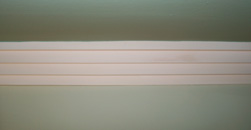Name Here  A = 7.25". B = 4.25". C = 120"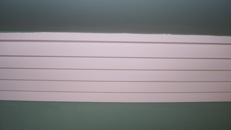Name Here  A = 7.25". B = 4.25". C = 120"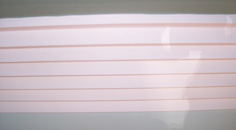Name Here  A = 7.25". B = 4.25". C = 120"Name Here  A = 7.25". B = 4.25". C = 120"Name Here  A = 7.25". B = 4.25". C = 120"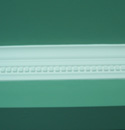Name Here  A = 7.25". B = 4.25". C = 120"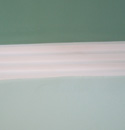Name Here  A = 7.25". B = 4.25". C = 120"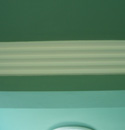Name Here  A = 7.25". B = 4.25". C = 120"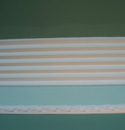Name Here  A = 7.25". B = 4.25". C = 120"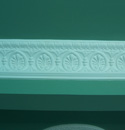Name Here  A = 7.25". B = 4.25". C = 120"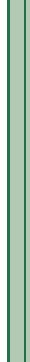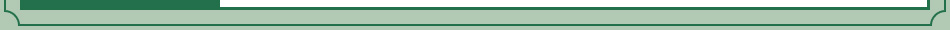Website by MWDUK.com